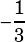In the xy-coordinate plane, the slope of line l is 3 and its y-intercept is 2. What is the equation of the line perpendicular to l that intersects l at its y-intercept?

The slope of the perpendicular line must be. Its y-intercept, of course, will still be 2. And when we use slope-intercept form (AKA y = mx + b form), the slope and the y-intercept are all we need to write the equation!